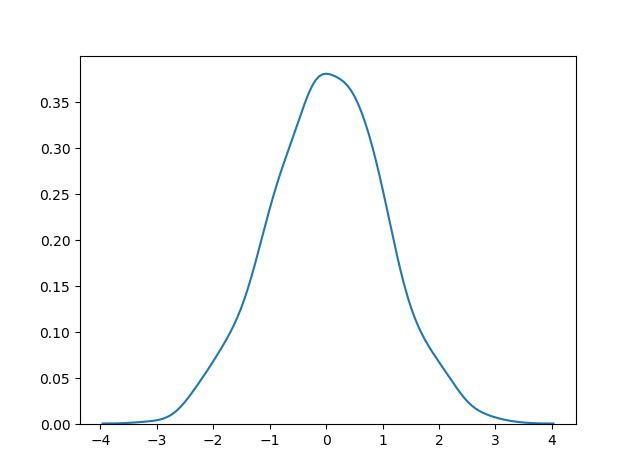# Normal (Gaussian) Distribution

## Normal Distribution

The Normal Distribution is one of the most important distributions.

It is also called the Gaussian Distribution after the German mathematician Carl Friedrich Gauss.

It fits the probability distribution of many events, eg. IQ Scores, Heartbeat etc.

Use the `random.normal()` method to get a Normal Data Distribution.

It has three parameters:

`loc` - (Mean) where the peak of the bell exists.

`scale` - (Standard Deviation) how flat the graph distribution should be.

`size` - The shape of the returned array.

### Example

Generate a random normal distribution of size 2x3:

from numpy import random

x = random.normal(size=(2, 3))

print(x)
Try it Yourself »

### Example

Generate a random normal distribution of size 2x3 with mean at 1 and standard deviation of 2:

from numpy import random

x = random.normal(loc=1, scale=2, size=(2, 3))

print(x)
Try it Yourself »

## Visualization of Normal Distribution

### Example

from numpy import random
import matplotlib.pyplot as plt
import seaborn as sns

sns.distplot(random.normal(size=1000), hist=False)

plt.show()

### ResultTry it Yourself »

Note: The curve of a Normal Distribution is also known as the Bell Curve because of the bell-shaped curve.